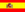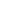home | más chusmeríos | otros temas de Física | lecciones del maestro Ciruela | tonterías | @NO ME SALEN
THEORETICAL NOTES AND EXCERCISES OF PHYSICS AND BIOPHYSICSMiscellany
Passage units
MULTIPLICATION BY ONE (NOT ANY ONE)

You have to remember two things: a number multiplied by one, results in the same number, and the result of a number divided by the same number, is one. Here you have some examples:

4 . 1 = 4 ;     37 . 1 = 37 ;     0,36 . 1 = 0,36 ;    a . 1 = a

8 / 8 = 1 ;     59 / 59 = 1 ;     0,24 / 0,24 = 1 ;     e / e = 1

Knowing this, we can say:

1 día / 24 h = 1

Because both numerator and denominator are the same. These quotients equal to one are non-innocent: we can use the to remove the units we want to disappear (and the units we want to appear, do so). Before starting, let me write in each conversion, the "ones" you should use:

Example 1) Convert 20 m/s to km/h
 1 km =    1 , 3.600 s =     1 1.000 m 1 h
Then I multiply the magnitude I want to convert by one, in this case, twice.
 20 m . 1 km . 3.600 s = s 1.000 m 1 h

Then, after cancelling those units on the numerator and the ones in the denominator (and after doing some little calculations), I have...

20 m/s = 72 km/h

Example 2) Convert 3.500 km/h² to m/s²

As one kilometer (kilo means thousand) is equal to 1000 meters,

1 km = 1.000 m                   (una igualdad)

And as one hour is the same as 3600 seconds, in an hour times un hour (h2) there are 3600 seconds times 3600 seconds,

1h² = 12.960.000 s²          (otra igualdad);

I assemble "the non-innocent ones"

 1.000 m =    1 1 h² =    1 1 km 12.960.000 s²
Now I multiply the magnitudes I want to convert by these "ones".
 3.500 km . 1.000 m . 1 h² = h² 1 km 12.960.000 s²

Now I cancell those units that appear on the numerator and in the denominator, and after some calculations, I have...

3.500 km/h² = 0,27 m/s²

Example 3) Convert 0,7 kgf/cm to N/m

As everyone knows, 1 kgf = 10 N, y 1m = 100 cm. Knowing this, I assemble the fractions I need:

 10 N =    1 , 100 cm =     1 1 kgf 1m
I use this to multiply the magnitude I want to converts; like, they value is equal to one, I´m not going to alter their value:
 0,7 kgf . 10 N . 100 cm = cm 1 kgf 1m

I operate a little and...

0,7 kgf/cm = 700 N/m

Example 4) Convert 60.000  J/day to W
1 W (1 Watt) is, by definition, 1 J/s.
 1 W =    1 1 W . s =    1 1 día =    1 1 J/s 1 J 86.400 s
(I suppose that you remember that what is dividing in the denominator, is in fact, multiplying in the numerator).
 60.000  J 1 W . s 1 day = day 1 J 86.400 s

chipsy fo´ the birdsy

60.000  J/day = 0,694  W

This is the method I usually teach in my class.Some rights reserved. Reproduction allowe by quoting the source. Translated by Santi Pisci. Last Updated jul-15. Buenos Aires, Argentina.